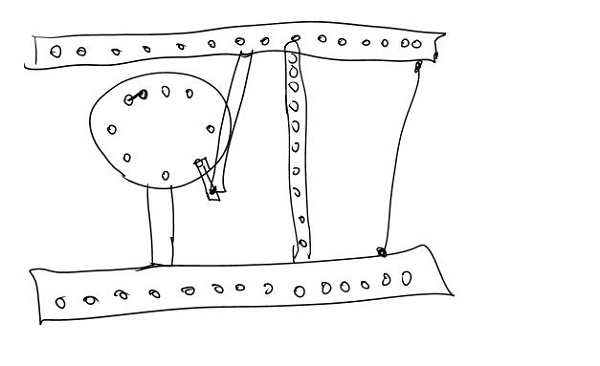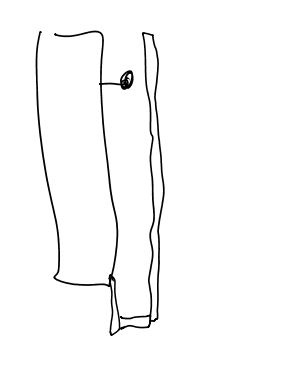# Launching Mechanisms Pt. 2

Tags: mechanical, innovate, design, and think
Personhours: 6
Launching Mechanisms Pt. 2 By Ethan

### Task: To improve upon launching mechanism designs

##### Catapult

First and foremost, we now have one completely functional, terrifying, catapult. The motor mechanism is cannibalized from our sister team's attempt at a catapult, which broke apart on testing.##### Flywheel
So, while we don't have a functional flywheel as of yet, we have done the math in order to get it up and working on the first try.

For reference, we need to launch the ball ~1 meter, the frequency of the motor is 2.53 s^-1, and the radius of our gear is 2.65cm. ``` velocityfinal^2 = velocityinitial^2 + 2*acceleration*change in y velocityfinal = sqrt(2*9.8m/s^2*1m) velocityfinal = sqrt(19.6m^2/s^2) velocityfinal = 4.427 m/s actual final velocity = 4.427 * 1.25 = 5.534m/s actual final velocity = 2*pi*radius*frequency (5.534m/s)/(2*pi*2.53s^-1) = .348m gear ratio = gear1radius/gear2radius = 2.65/.348 = 1:7.615 However, for simplicity, our needed gear ratio is 1:8. ```

### Reflections

We should advance in building our attachments - if we go to Arkansas, we have <6 practices to go. And, from now on, we need to do the math behind them so we know we're doing them right.

Date | October 31, 2016